awas58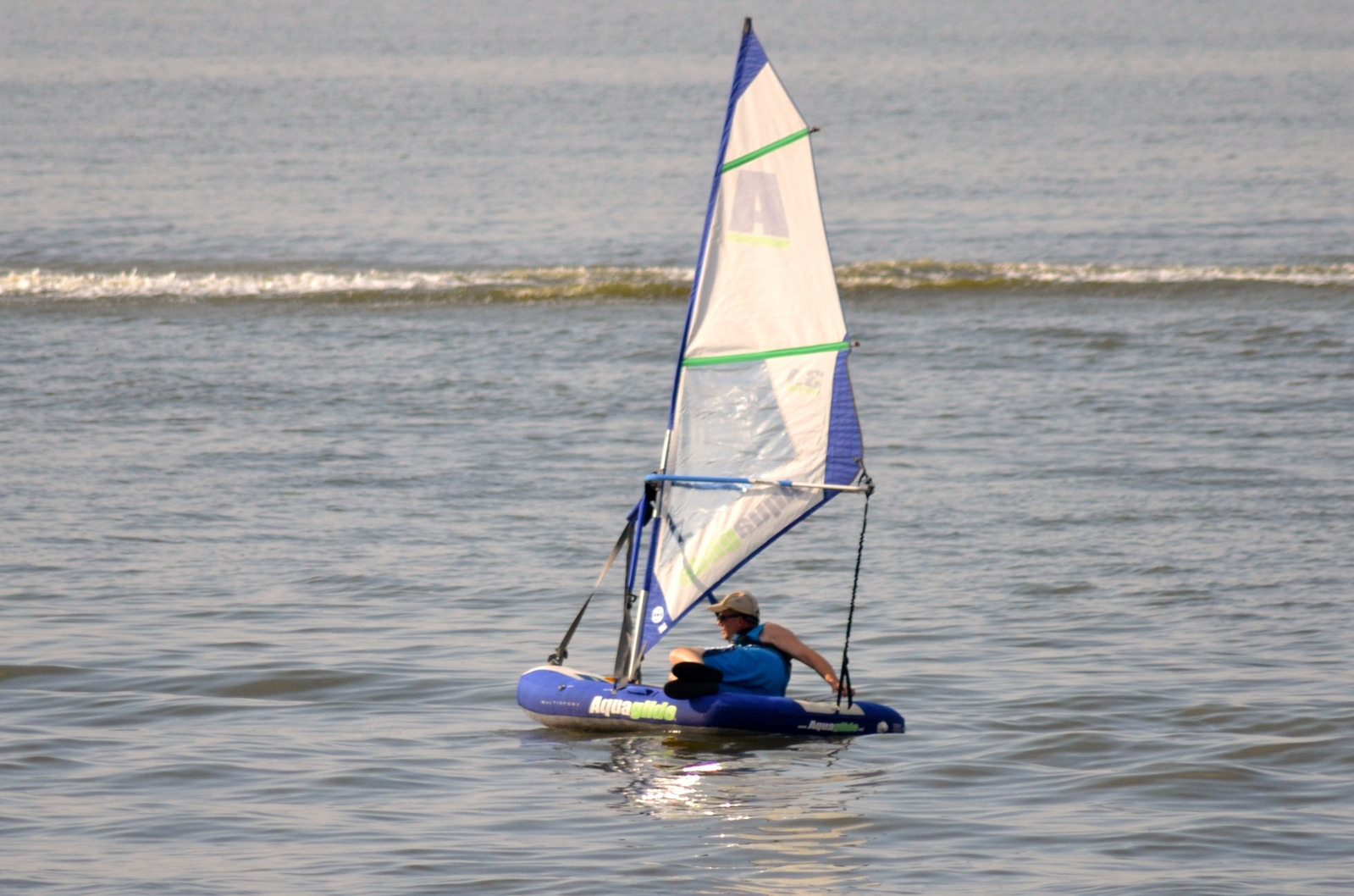User #6911 - awas58 Author awas58 Title #192104 Caption Session Exposure 1/1600s f/5.6 ISO 400 300mm White balance Automatic Exposure program & metering mode not defined, Pattern Model NIKON D3100 Lens Software Microsoft Windows Live Photo Gallery14.0.8051.1204 Photo created at 2012-06-24 17:49:36 Uploaded 2013-01-30 10:19:09 Geoposition LAT : LON : ALT :
 Attractiveness 0 Views 0
Share
Copy the selected link in your clipboard by using keyboard shortcuts CTRL + C and paste it in your target location using CTRL + V
(ID:6911)
See
Comments and rating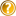Rate photo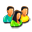Rating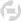5.8 Composition5.8 Technique5.8 Artistry5.8 Conception5.8 Emotion5.8 Your rating Common rating 5.8 (Rating amount : 4)Add comment(Maximum characters: 1024) You have 1024 characters left. Comment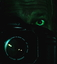D6982_Hornet 2013-02-02 01:32:50 :)))))))))))))))))))))))))))))))))))))))))))))))))))))))))))))))))))))))))))))))))))))))))))))))))))))))))))))))))))))))))))))))))))))))))))))))))))))))))))))))))))))))))))))))))))))))))))))))))))))))))))))))))))))))))))))))))))))))))))))))))))))))))))))))))))))))))))))))))))))))))))))))))))))))))))))))))))))))))))))))))))))))))))))))))))))))))))))))))))))))))))))))))))))))))))))))))))))))))))))))))))))))))))))))))))))))))))))))))))))))))))))))))))))))))))))))))))))))))))))))))))))))))))))))))))))))))))))))))))))))))))))))))))))))))))))))))))))))))))))))))))))))))))))))))))))))))))))))))))))))))))))))))))))))))))))))))))))))))))))))))))))))))))))))))))))))))))))))))))))))))))))))))))))))))))))))))))))))))))))))))))))))))))))))))))))))))))))))))))))))))))))))))))))))))))))))))))))))))))))))))))))))))))))))))))))))))))))))))))))))))))))))))))))))))))))))))))))))))))))))))))))))))))))))))))))))))))))))))))))))))))))))))))))))))))))))))))))))))))))))))))))))))))))))))))))))))))))))))))))))))))))))))))))))))))))))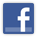Visit us on Facebook or Google plus and learn more about events and competitions connected with our portal before others.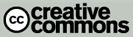Our service allows for including a copyright notice in each photograph based on the Creative Commons solutions. Click on the logo to learn more about the international Creative Commons project.